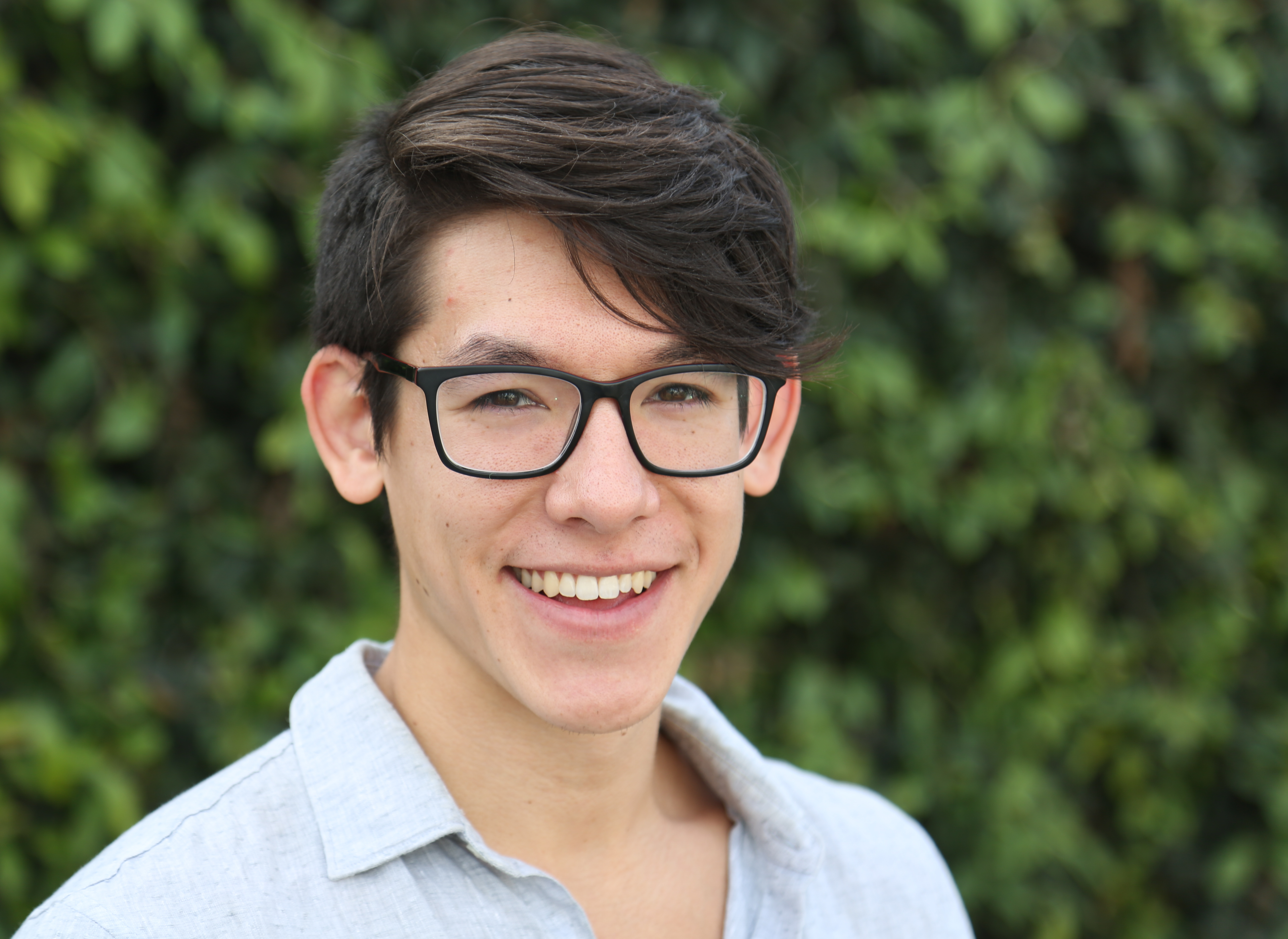## Algebra 1&2

#### Session length: 60 minutes#### 1-1 Instructor: Pieter Zenner

###### Harvard University

Algebra 1: Topics include solving and describing diverse equations, linear equations and inequalities, systems of equations, exponents and exponential functions, quadratic and polynomial functions, and data analysis.
Algebra 2: Students build on their Algebra foundation, comparing commonalities among families of functions including linear, quadratic, polynomial, radical, exponential, logarithmic, rational, and trigonometric functions.